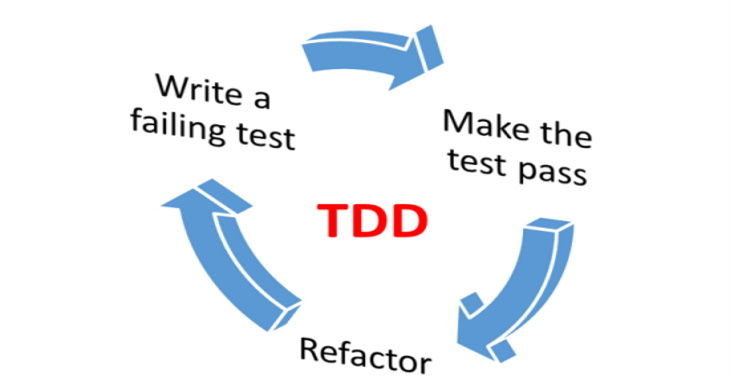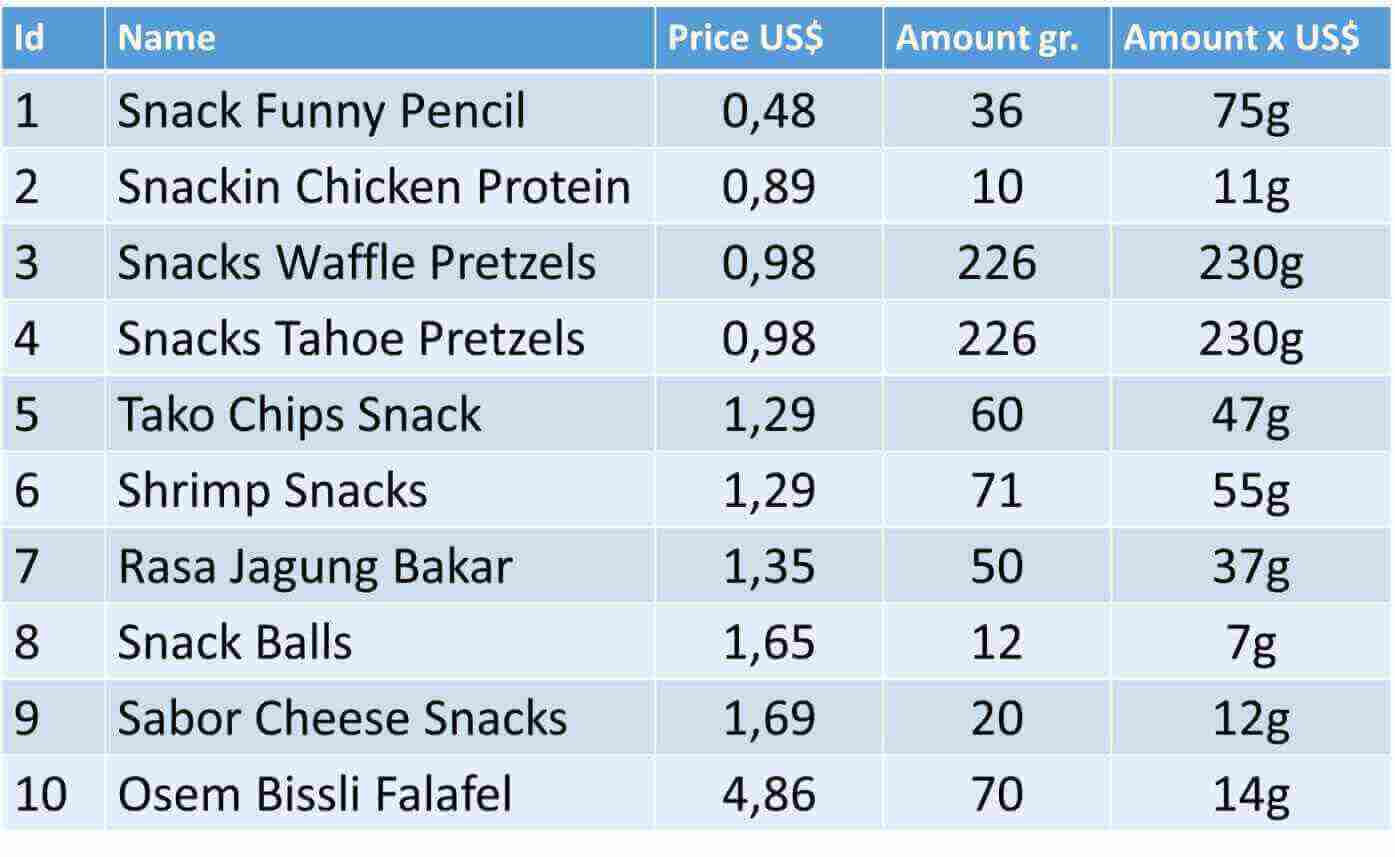Moises Gamio Software Engineer. Comprehensive experience in all phases of the software development lifecycle for several economic sectors.

# Learning Test-Driven DevelopmentTest-driven development (TDD) is a development approach that emphasizes writing a test before writing the necessary code and then refactoring the code to optimize it.

## Problem

Optimize a basket in online purchases means fill a basket with the most valuable goods under a given budget.

Imagine that we have a budget of 4 US\$ and we want to buy the most valuable snacks from the following table:But who decides if a product is more valuable than another one? Well, this depends on every business. It could be an estimation based on quantitative or qualitative analysis. For instance, for our solution, we choose a quantitative approach based on which product gives us more grams per every dollar invested.

## Optimize a basket in online purchases: Solution

To implement our algorithm to optimize a basket in online purchases, we use the Red-Green Refactor technique, which is the basis of test-drive-development (TDD). Firstly, in every assumption, we will write a test and see if it fails. Secondly, we write the code that implements only that test and sees if it succeed, then we can refactor the code to make it better. Finally, we continue with another assumption and repeat the previous steps until the algorithm is successfully implemented for all tests.

To generalize the concept of “the most valuable product” we assign a value to every product. Our algorithm receives two parameters: an array 2-D which includes [product-id][price][value] and the budget.

Assumption #1 – Given an array of products ordered by value, return the most valuable products

We start defining a java test creating a new BasketOptimized class. For naming variables, you can read clean code.

``````1
2
3
4
5
6
7
8
9
10
11
12
13
14
15
16
17
18
19
20
21
22
23
24
25
26
27
28

@Before
public void setup() {
}

@Test
public void productsOrderedByValue () {

double[][] myProducts = new double[][] {
{1, 0.98, 230},
{2, 0.98, 230},
{3, 0.48, 75},
{4, 1.29, 55},
{5, 1.29, 47},
{6, 4.86, 14},
{7, 1.69, 12},
};

double[][] mostValueableProducts =
assertEquals(590d,
Arrays.stream(mostValueableProducts).
mapToDouble(arr -> arr).sum(),0);
}
}
``````

The first time, it should fail because the fill method doesn’t exist. Then we need to create an easy implementation to pass the test: the sum of the values must be equal to 590 because this represents all selected products which its prices sum less or equal than 4.

Now, we proceed to implement the fill method.

``````1
2
3
4
5
6
7
8
9
10
11
12
13
14
15
16
17
18
19
20
21
22

public double[][] fill(double[][] myProducts, double budget) {

int len = myProducts.length;
double[][] mostValueableProducts =  new double[len];

double sum = 0;
for (int idx=0; idx < len; idx++) {
sum = sum + myProducts[idx]; //price
if (sum <= budget) {
mostValueableProducts[idx] =
myProducts[idx]; //id
mostValueableProducts[idx] =
myProducts[idx]; //price
mostValueableProducts[idx] =
myProducts[idx]; //value
}
}
return mostValueableProducts;
}
}
``````

Assumption #2 – Given an array of products not ordered by value, return the most valuable products

In this case we pass a not ordered array, so we can see that our new test will fail.

``````1
2
3
4
5
6
7
8
9
10
11
12
13
14
15
16
17
18
@Test
public void productsNotOrderedByValue () {

double[][] myProducts = new double[][]{
{1, 0.98, 230},
{2, 1.29, 47},
{3, 1.69, 12},
{4, 1.29, 55},
{5, 0.98, 230},
{6, 4.86, 14},
{7, 0.48, 75}
};

double[][] mostValueableProducts
assertEquals(590d, Arrays.stream(mostValueableProducts)
.mapToDouble(arr -> arr).sum(), 0);
}
``````

We realize that we need to order the array by value because we want the most valuable products, so its time to refactor our algorithm. What we need to do is to sort our input array.

``````1
2
3
4
5
6
public double[][] fill(double[][] myProducts, double budget) {

Arrays.sort(myProducts, Collections.reverseOrder(
Comparator.comparingDouble(a -> a)));
int len = myProducts.length;
double[][] mostValueableProducts =  new double[len];
``````

Then we can see our two test cases were successful.

### Calculate combinations

Assumption #3 – Given an array of products, we need to obtain the most valuable products from all possible combinations of the products

Imagine the following escenario:

``````1
2
3
4
5
6
7
8
9
10
11
12
13
14
15
double[][] myProducts = new double[][] {
{1, 0.98, 230},
{2, 0.51, 30},
{3, 0.49, 28},
{4, 1.29, 55},
{5, 0.98, 230},
{6, 4.86, 14},
{7, 0.48, 75},
};

.fill(myProducts, 4);
assertEquals(590d,
Arrays.stream(mostValueableProducts)
.mapToDouble(arr -> arr).sum(),0);
``````

The test is expecting a result of 590 which corresponds to the final price of 3,73US\$ (0.98+0.98+0.48+1.29). Once the algorithm sort by value the input array, we have the following result:

``````1
2
3
4
5
6
7
[1.0, 0.98, 230.0]
[5.0, 0.98, 230.0]
[7.0, 0.48, 75.0]
[4.0, 1.29, 55.0]
[2.0, 0.51, 30.0]
[3.0, 0.49, 28.0]
[6.0, 4.86, 14.0]
``````

But here we realize the exists another combination of products which give us the most valuable products: 230+230+75+30+28 = 593 which corresponds to the final price of 3,44US\$. Then we need to refactor our code to calculate all combinations (subsets) and return the most valuable products under a budget of 4 US\$.

The subsets can be represented by all the binary options from 0 to 7 (the array size).

``````1
2
3
4
5
6
7
0000 = {}
0001 = { {1, 0.98, 230} }
0010 = { {2, 0.51, 30} }
0011 = { {1, 0.98, 230}, {2, 0.51, 30} }
.
.
.
``````

We build a hashMap to store all combinations and the sum of its values. Finally, we return the first element of the hashMap ordered by value

``````1
2
3
4
5
6
7
8
9
10
11
12
13
14
15
16
17
18
19
20
21
int len = myProducts.length;
int numIterations = (int) Math.pow(2, myProducts.length);

Map<double[][], Double> combinations = new HashMap<>();

for (int idx=0; idx<numIterations; idx++){
double[][] combx = new double[len][];
double sumPrice = 0;
double sumValue = 0;
int i = 0;
for (int idx2=0; idx2<len; idx2++) {
if ((idx & (int) Math.pow(2, idx2)) == 0) {
combx[i++] = myProducts[idx2];
sumPrice = sumPrice + myProducts[idx2];
sumValue = sumValue + myProducts[idx2];
}
}
if (combx.length > 0 && sumPrice <= budget) {
combinations.put(combx, Double.valueOf(sumValue));
}
}
``````

You can see the complete solution explained in detail and all test cases in this link